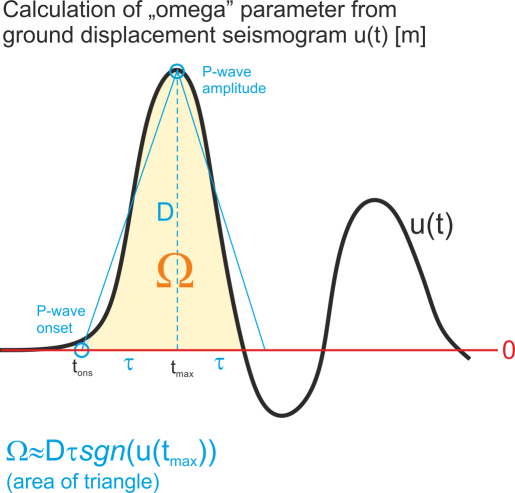# hybridmt

## What is the magnitude limit for application of fociMT or hybridMT algorithms?

The fociMT algorithm is point-source moment tensor inversion and the fault rupture processes (e.g. directivity or spatial changes in elementary moment tensors) are NOT considered. As such, the algorithm is valid for events with simple source time functions that can be represented by a single pulse. Therefore, it is suggested to use the algorithm for events with moment magnitudes not exceeding Mw 4.

## Should focal mechanisms (or moment tensors) I intend to refine altogether with hybridMT technique be similar?

No. There is no requirement that input data come from earthquakes displaying similar focal mechanism. The hybridMT refinement method is NOT based on empirical Green’s function technique, where this requirement is imposed. Actually, the larger the variability of focal mechanism, the better refinement of seismic moment tensors is generally expected.

## Is your method for refining seismic moment tensor based on empirical Green’s function technique?

Shortly speaking, no. The moment tensor refinement method used in hybridMT algorithm is NOT using the concept of empirical Green’s function technique, although the refinement of seismic moment tensors is also achieved by suppressing the propagation effects (similarly to EGF method). As such, none of EGF assumptions (i.e. similarity of focal mechanisms forming the cluster) is not relevant for hybridMT refinement.

## What is the “omega” parameter in the focimt input file and how to calculate it?“omega” parameter which is a part of ASCII input file is basically the area below the first P-wave ground displacement pulse (in time domain) or equivalently the spectral level taken from the amplitude spectrum of ground displacement pulse. The unit of “omega” parameter is [m * s] (meter times second), and it comes from the fact that we multiply the amplitude of first P-wave pulse using ground displacement seismogram in [m] by the half of duration of the first P-wave pulse in [s]. Regardless of whether time or frequency domain was used to extract the “omega” value, the parameter should contain the sign information. The sign of “omega” is in accordance with the seismological convention, i.e. it is positive for the positive first P-wave ground displacement amplitude (i.e. ground motion AWAY from the seismic source) and negative otherwise (ground motion TOWARDS the seismic source).

In case of simple impulsive source time functions it is typically not necessary to calculate the integral of ground displacement first P-wave pulse. Instead, the amplitude D (cf. picture) including sign information of first P-wave pulse may be taken from ground displacement seismogram (the seismogram should be calibrated to [m]) and multiplied by the the rise time (i.e. time interval between the P-wave onset and P-wave amplitude, t_max-t_ons in seconds [s]). In this case, the integral of the pulse is simply replaced by the area of the triangle.